# NCERT Exemplar Class 11 Chemistry Solutions for Chapter 11 - The P Block Elements

## NCERT Exemplar Solutions Class 11 Chemistry Chapter 11 – Free PDF Download

NCERT Exemplar Solutions for Chemistry Class 11 Chapter 11 The p-Block Elements are provided here for students to prepare well for CBSE Class 11, engineering and medical entrance examinations. In order to understand the topic thoroughly, it is essential for the students to get well-versed in this exemplar solution. The NCERT Exemplar Class 11 Chemistry Chapter 11 PDF includes The p-Block Elements Class 11 questions from the NCERT Exemplar book, along with The p-Block Elements questions and answers from question papers and sample papers, and other problems on p-Block Elements.

Studying this NCERT Exemplar thoroughly will help you in grasping the concepts, and you can make p-Block Elements Class 11 important notes which will come in handy during the revision of the chapter. P-block elements is one of the most important topics of Chemistry. The basics of p-block elements are taught in Class 11, and advanced topics are taught in Class 12. After studying this chapter, students will be able to appreciate the general trends in the Chemistry of p-block elements. They will also learn more about the Chemistry of some important compounds of boron, carbon and silicon.

### Download the PDF of the NCERT Exemplar for Class 11 Chemistry Chapter 11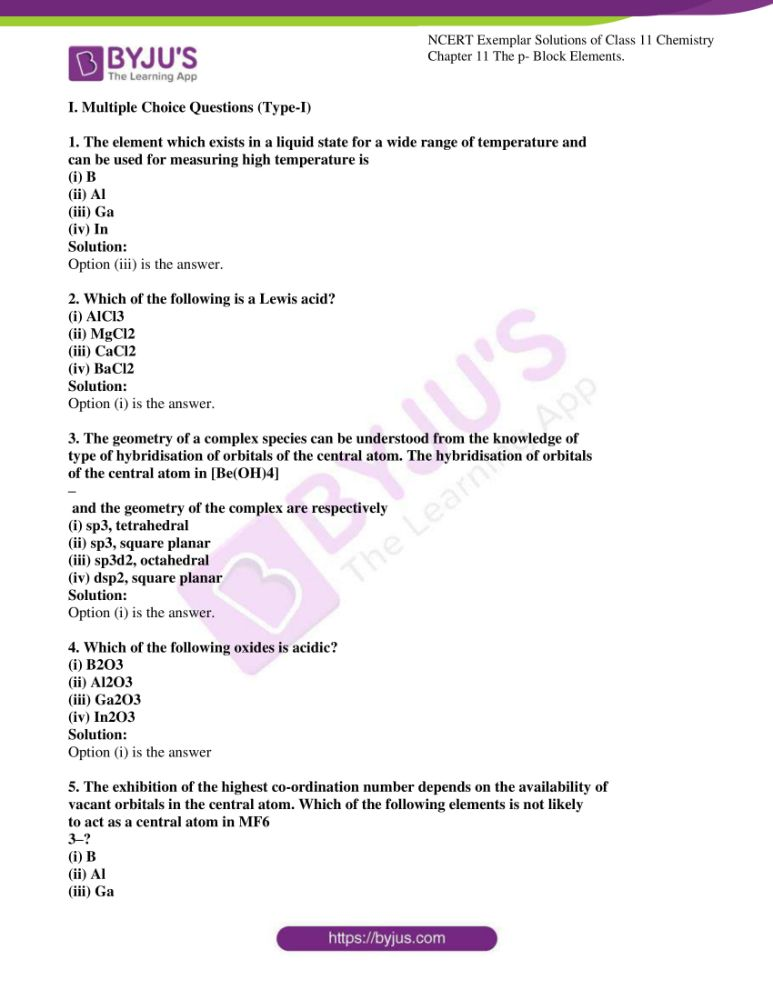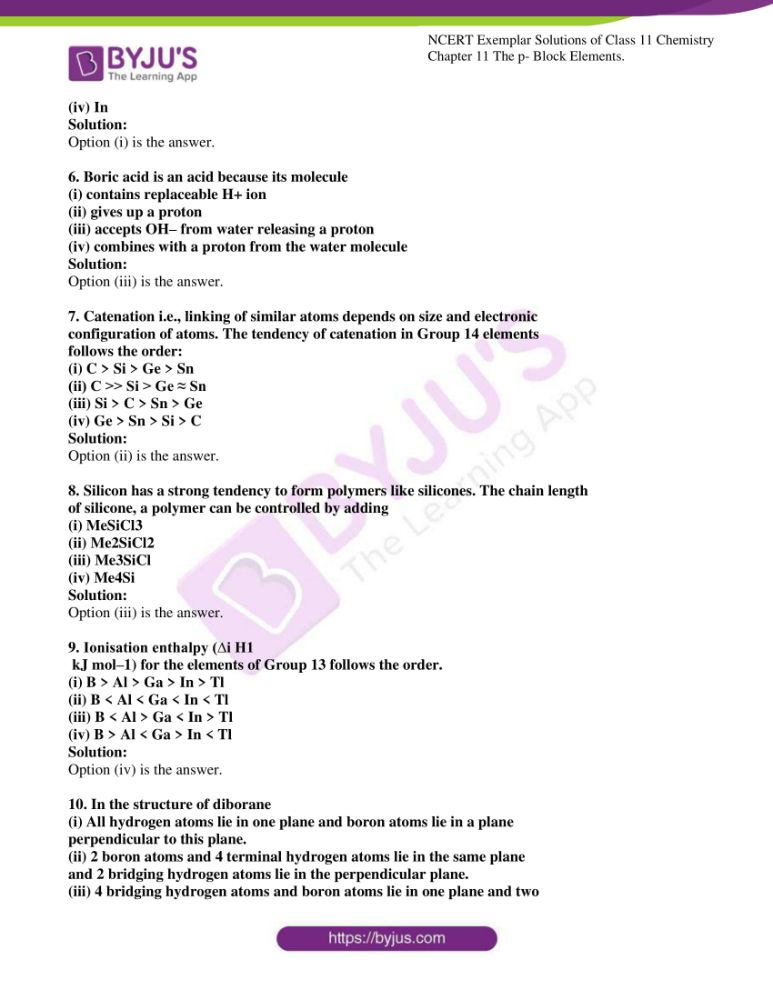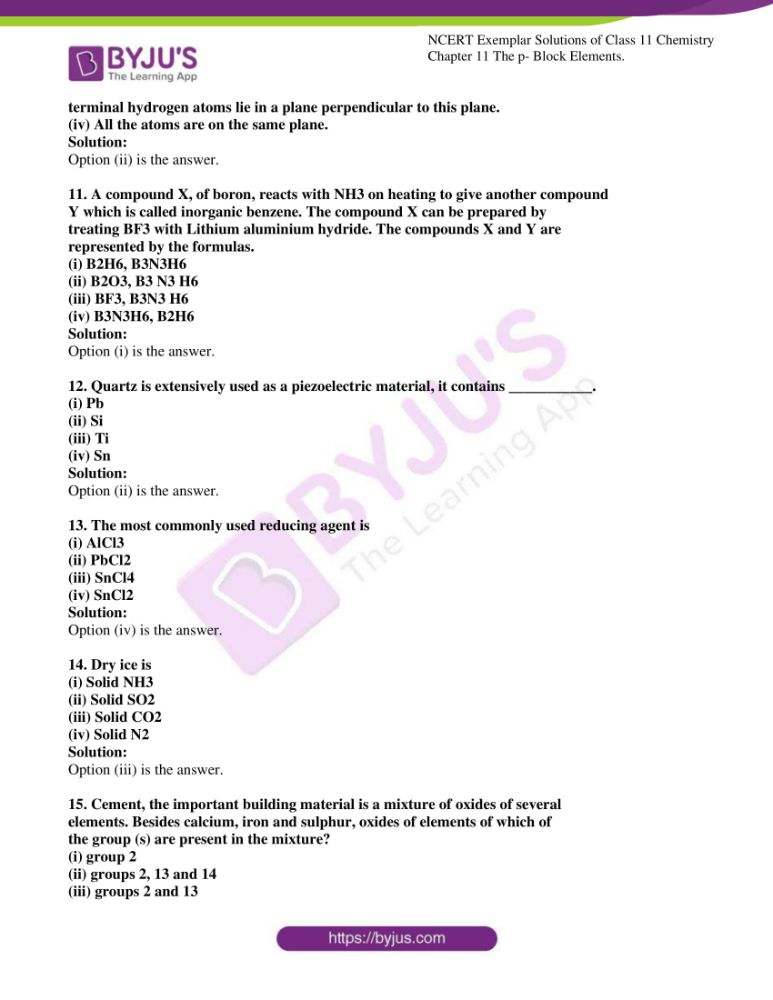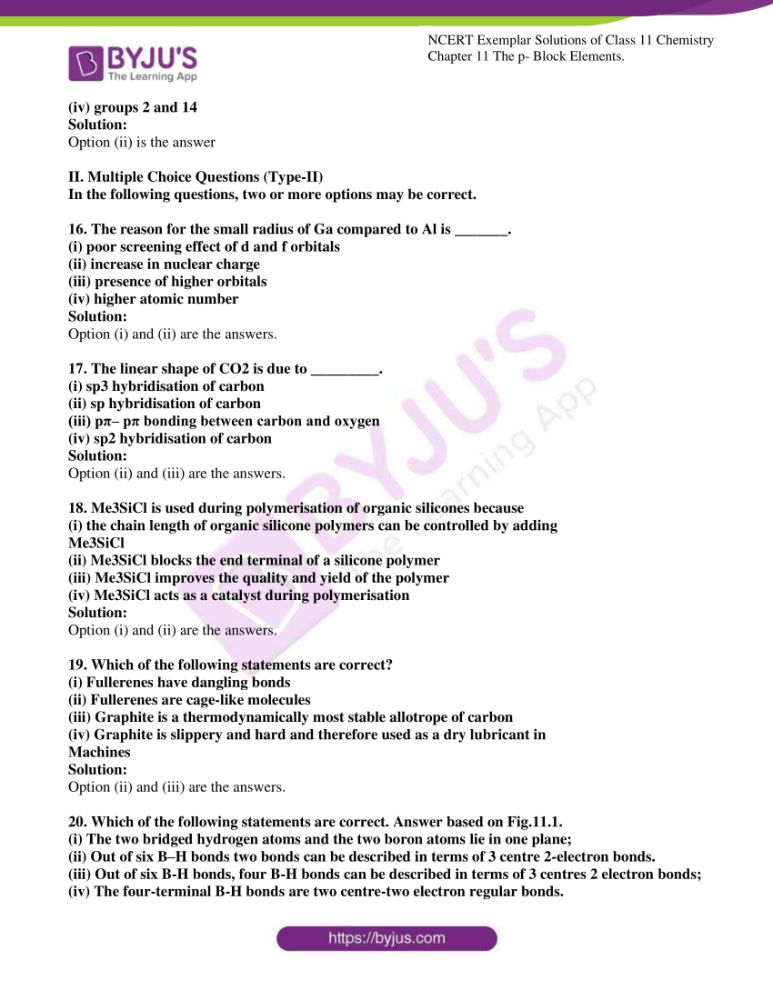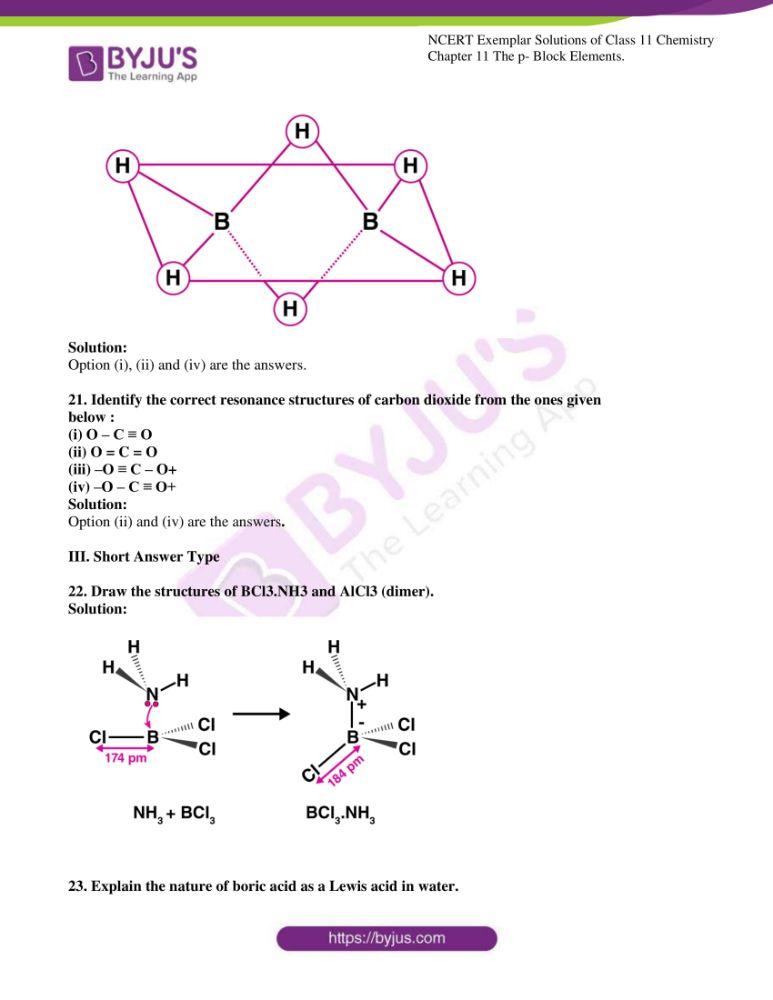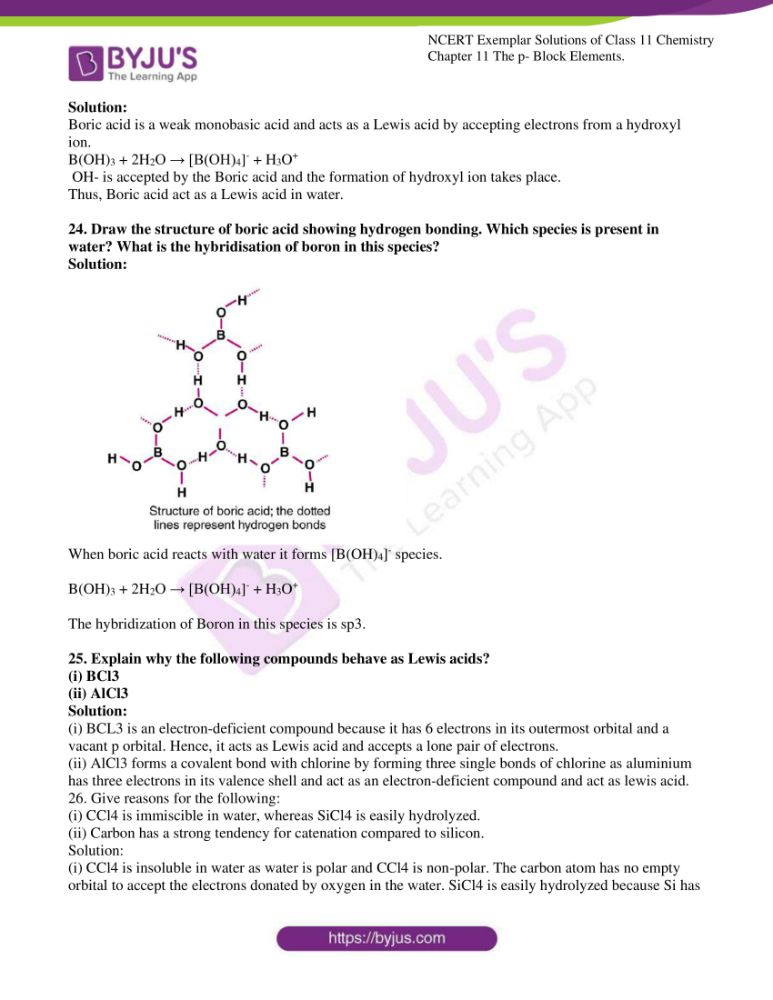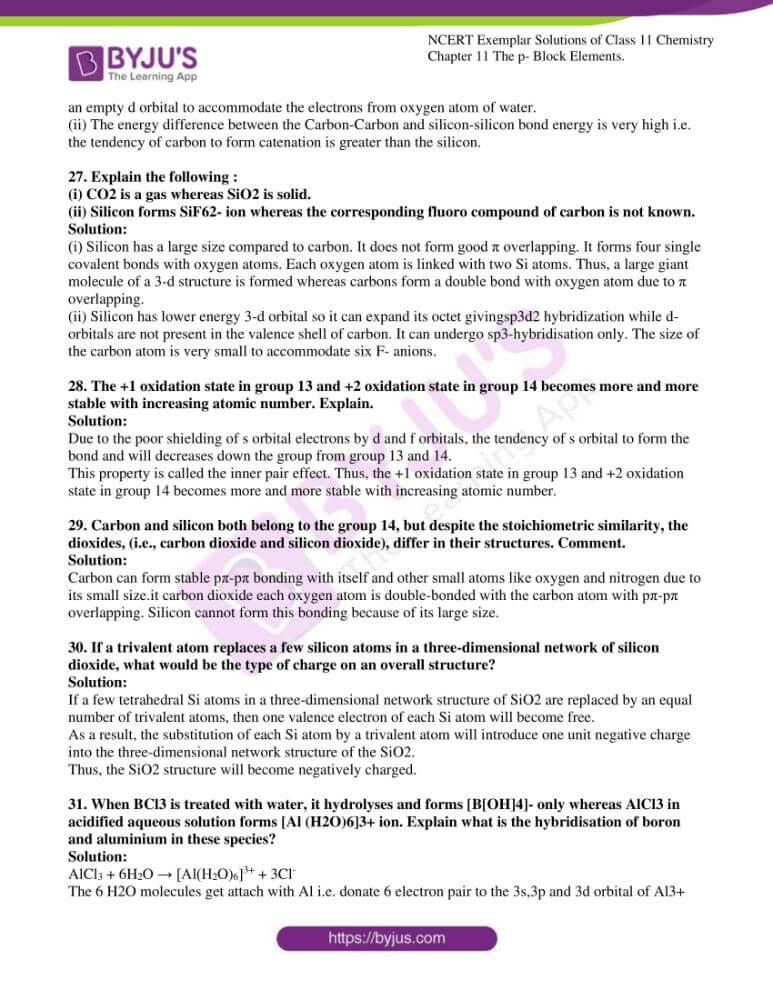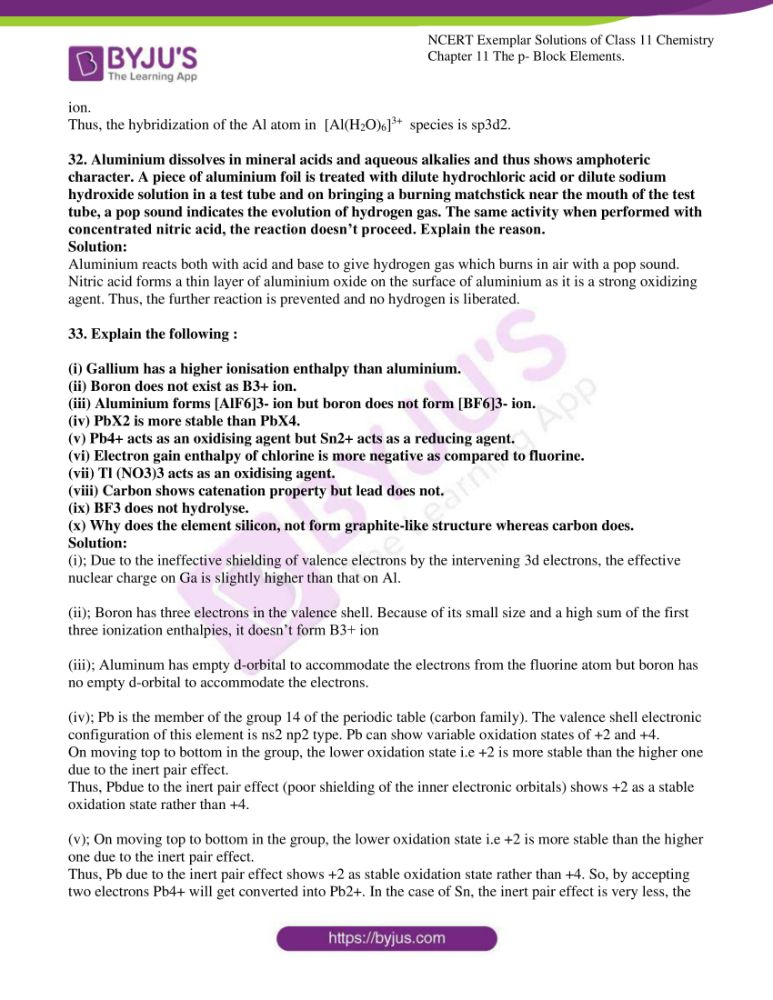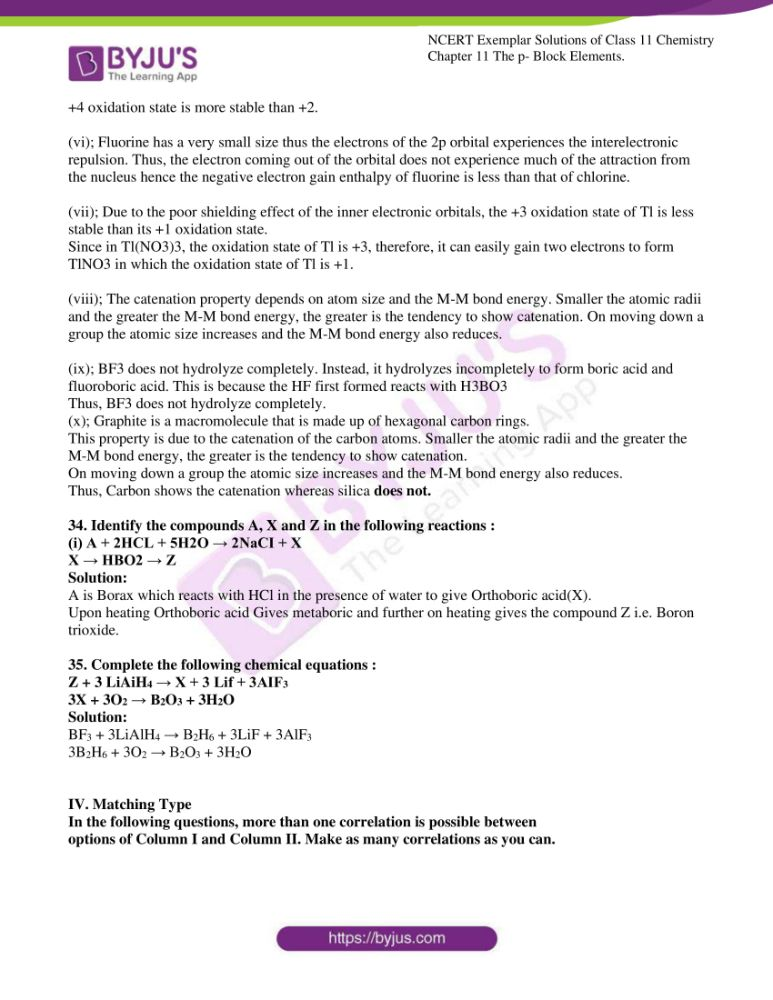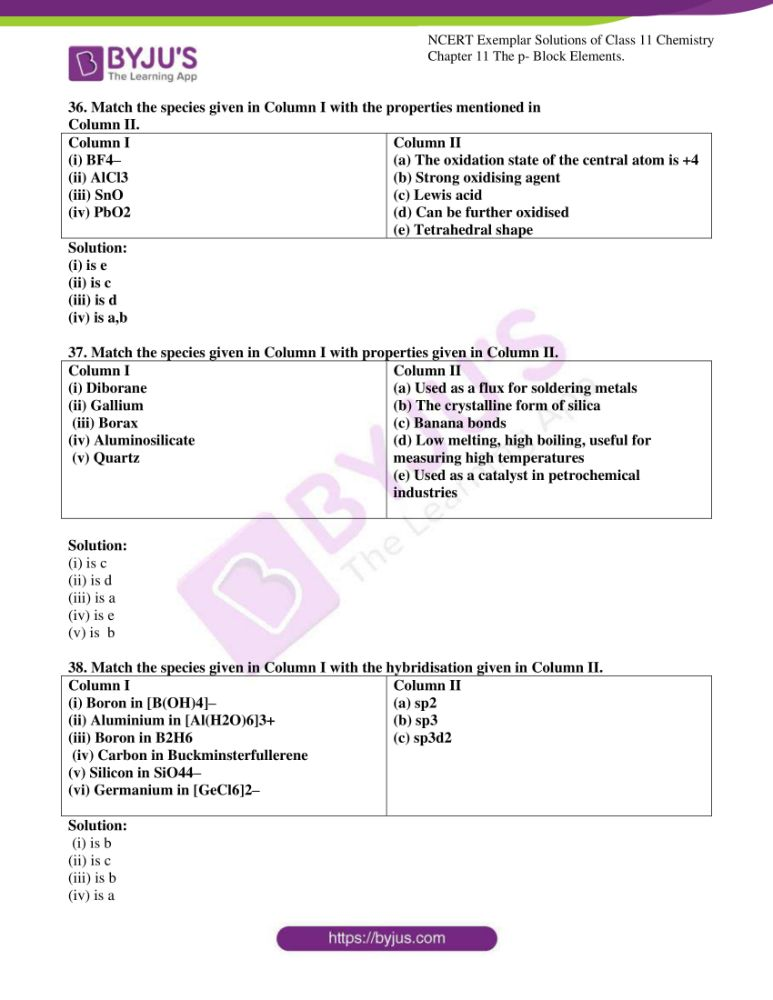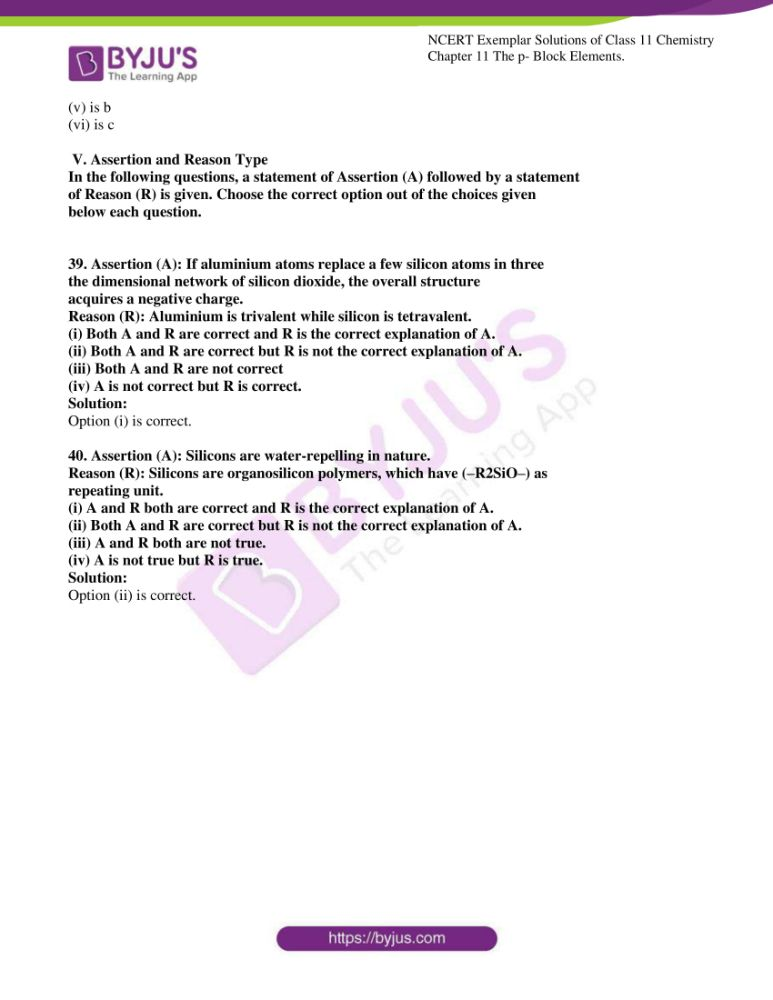### Access Answers to the NCERT Exemplar for Class 11 Chemistry Chapter 11

I. Multiple Choice Questions (Type-I)

1. The element which exists in a liquid state for a wide range of temperature and

can be used for measuring high temperature is

(i) B

(ii) Al

(iii) Ga

(iv) In

Solution:

2. Which of the following is a Lewis acid?

(i) AlCl3

(ii) MgCl2

(iii) CaCl2

(iv) BaCl2

Solution:

3. The geometry of a complex species can be understood from the knowledge of

type of hybridisation of orbitals of the central atom. The hybridisation of orbitals

of the central atom in [Be(OH)4]

and the geometry of the complex are respectively

(i) sp3, tetrahedral

(ii) sp3, square planar

(iii) sp3d2, octahedral

(iv) dsp2, square planar

Solution:

4. Which of the following oxides is acidic?

(i) B2O3

(ii) Al2O3

(iii) Ga2O3

(iv) In2O3

Solution:

5. The exhibition of the highest co-ordination number depends on the availability of

vacant orbitals in the central atom. Which of the following elements is not likely

to act as a central atom in MF6

3–?

(i) B

(ii) Al

(iii) Ga

(iv) In

Solution:

6. Boric acid is an acid because its molecule

(i) contains replaceable H+ ion

(ii) gives up a proton

(iii) accepts OH– from water releasing a proton

(iv) combines with a proton from the water molecule

Solution:

7. Catenation i.e., linking of similar atoms depends on size and electronic

configuration of atoms. The tendency of catenation in Group 14 elements

follows the order:

(i) C > Si > Ge > Sn

(ii) C >> Si > Ge ≈ Sn

(iii) Si > C > Sn > Ge

(iv) Ge > Sn > Si > C

Solution:

8. Silicon has a strong tendency to form polymers like silicones. The chain length

of silicone, a polymer can be controlled by adding

(i) MeSiCl3

(ii) Me2SiCl2

(iii) Me3SiCl

(iv) Me4Si

Solution:

9. Ionisation enthalpy (∆i H1

kJ mol–1) for the elements of Group 13 follows the order.

(i) B > Al > Ga > In > Tl

(ii) B < Al < Ga < In < Tl

(iii) B < Al > Ga < In > Tl

(iv) B > Al < Ga > In < Tl

Solution:

10. In the structure of diborane

(i) All hydrogen atoms lie in one plane and boron atoms lie in a plane

perpendicular to this plane.

(ii) 2 boron atoms and 4 terminal hydrogen atoms lie in the same plane

and 2 bridging hydrogen atoms lie in the perpendicular plane.

(iii) 4 bridging hydrogen atoms and boron atoms lie in one plane and two

terminal hydrogen atoms lie in a plane perpendicular to this plane.

(iv) All the atoms are on the same plane.

Solution:

11. A compound X, of boron, reacts with NH3 on heating to give another compound

Y which is called inorganic benzene. The compound X can be prepared by

treating BF3 with Lithium aluminium hydride. The compounds X and Y are

represented by the formulas.

(i) B2H6, B3N3H6

(ii) B2O3, B3 N3 H6

(iii) BF3, B3N3 H6

(iv) B3N3H6, B2H6

Solution:

12. Quartz is extensively used as a piezoelectric material, it contains ___________.

(i) Pb

(ii) Si

(iii) Ti

(iv) Sn

Solution:

13. The most commonly used reducing agent is

(i) AlCl3

(ii) PbCl2

(iii) SnCl4

(iv) SnCl2

Solution:

14. Dry ice is

(i) Solid NH3

(ii) Solid SO2

(iii) Solid CO2

(iv) Solid N2

Solution:

15. Cement, the important building material is a mixture of oxides of several

elements. Besides calcium, iron and sulphur, oxides of elements of which of

the group (s) are present in the mixture?

(i) group 2

(ii) groups 2, 13 and 14

(iii) groups 2 and 13

(iv) groups 2 and 14

Solution:

II. Multiple Choice Questions (Type-II)

In the following questions, two or more options may be correct.

16. The reason for the small radius of Ga compared to Al is _______.

(i) poor screening effect of d and f orbitals

(ii) increase in nuclear charge

(iii) presence of higher orbitals

(iv) higher atomic number

Solution:

Option (i) and (ii) are the answers.

17. The linear shape of CO2 is due to _________.

(i) sp3 hybridisation of carbon

(ii) sp hybridisation of carbon

(iii) pπ– pπ bonding between carbon and oxygen

(iv) sp2 hybridisation of carbonSolution:

Option (ii) and (iii) are the answers.

18. Me3SiCl is used during polymerisation of organic silicones because

(i) the chain length of organic silicone polymers can be controlled by adding

Me3SiCl

(ii) Me3SiCl blocks the end terminal of a silicone polymer

(iii) Me3SiCl improves the quality and yield of the polymer

(iv) Me3SiCl acts as a catalyst during polymerisationSolution:

Option (i) and (ii) are the answers.

19. Which of the following statements are correct?

(i) Fullerenes have dangling bonds

(ii) Fullerenes are cage-like molecules

(iii) Graphite is a thermodynamically most stable allotrope of carbon

(iv) Graphite is slippery and hard and therefore used as a dry lubricant in

Machines

Solution:

Option (ii) and (iii) are the answers.

20. Which of the following statements are correct. Answer based on Fig.11.1.

(i) The two bridged hydrogen atoms and the two boron atoms lie in one plane;

(ii) Out of six B–H bonds two bonds can be described in terms of 3 centre 2-electron bonds.

(iii) Out of six B-H bonds, four B-H bonds can be described in terms of 3 centres 2 electron bonds;

(iv) The four-terminal B-H bonds are two centre-two electron regular bonds.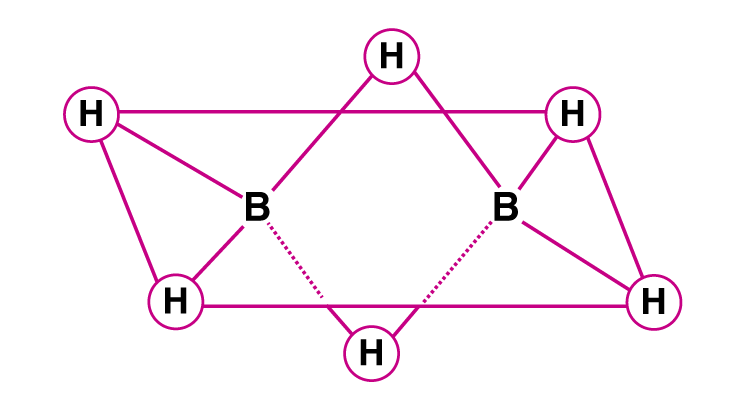Solution:

Option (i), (ii) and (iv) are the answers.

21. Identify the correct resonance structures of carbon dioxide from the ones given

below :

(i) O – C ≡ O

(ii) O = C = O

(iii) –O ≡ C – O+

(iv) –O – C ≡ O+

Solution:

Option (ii) and (iv) are the answers.

22. Draw the structures of BCl3.NH3 and AlCl3 (dimer).

Solution: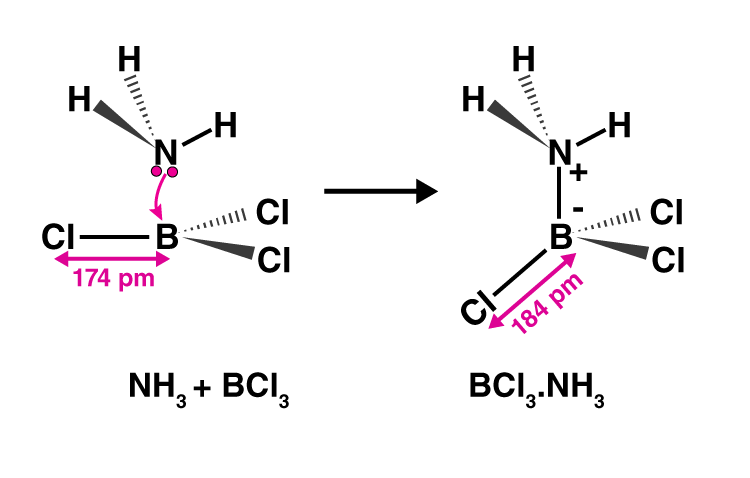23. Explain the nature of boric acid as a Lewis acid in water.

Solution:

Boric acid is a weak monobasic acid and acts as a Lewis acid by accepting electrons from a hydroxyl ion.

B(OH)3 + 2H2O → [B(OH)4] + H3O+

OH- is accepted by the Boric acid and the formation of hydroxyl ion takes place.

Thus, Boric acid act as a Lewis acid in water.

24. Draw the structure of boric acid showing hydrogen bonding. Which species is present in water? What is the hybridisation of boron in this species?

Solution: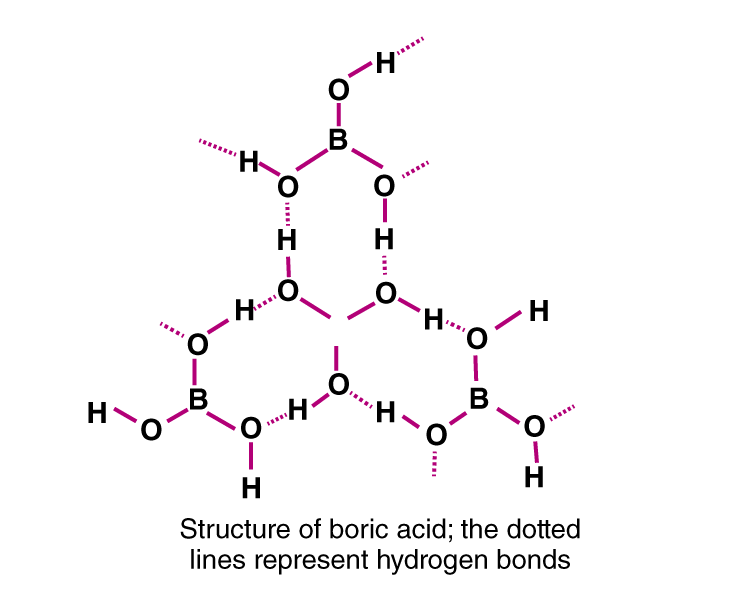When boric acid reacts with water it forms [B(OH)4] species.

B(OH)3 + 2H2O → [B(OH)4] + H3O+

The hybridization of Boron in this species is sp3.

25. Explain why the following compounds behave as Lewis acids?

(i) BCl3

(ii) AlCl3

Solution:

(i) BCL3 is an electron-deficient compound because it has 6 electrons in its outermost orbital and a vacant p orbital. Hence, it acts as Lewis acid and accepts a lone pair of electrons.

(ii) AlCl3 forms a covalent bond with chlorine by forming three single bonds of chlorine as aluminium has three electrons in its valence shell and act as an electron-deficient compound and act as lewis acid.

26. Give reasons for the following:

(i) CCl4 is immiscible in water, whereas SiCl4 is easily hydrolyzed.

(ii) Carbon has a strong tendency for catenation compared to silicon.

Solution:

(i) CCl4 is insoluble in water as water is polar and CCl4 is non-polar. The carbon atom has no empty orbital to accept the electrons donated by oxygen in the water. SiCl4 is easily hydrolyzed because Si has an empty d orbital to accommodate the electrons from oxygen atom of water.

(ii) The energy difference between the Carbon-Carbon and silicon-silicon bond energy is very high i.e. the tendency of carbon to form catenation is greater than the silicon.

27. Explain the following :

(i) CO2 is a gas whereas SiO2 is solid.

(ii) Silicon forms SiF62- ion whereas the corresponding fluoro compound of carbon is not known.

Solution:

(i) Silicon has a large size compared to carbon. It does not form good π overlapping. It forms four single covalent bonds with oxygen atoms. Each oxygen atom is linked with two Si atoms. Thus, a large giant molecule of a 3-d structure is formed whereas carbons form a double bond with oxygen atom due to π overlapping.

(ii) Silicon has lower energy 3-d orbital so it can expand its octet givingsp3d2 hybridization while d-orbitals are not present in the valence shell of carbon. It can undergo sp3-hybridisation only. The size of the carbon atom is very small to accommodate six F- anions.

28. The +1 oxidation state in group 13 and +2 oxidation state in group 14 becomes more and more stable with increasing atomic number. Explain.

Solution:

Due to the poor shielding of s orbital electrons by d and f orbitals, the tendency of s orbital to form the bond and will decreases down the group from group 13 and 14.

This property is called the inner pair effect. Thus, the +1 oxidation state in group 13 and +2 oxidation state in group 14 becomes more and more stable with increasing atomic number.

29. Carbon and silicon both belong to the group 14, but despite the stoichiometric similarity, the dioxides, (i.e., carbon dioxide and silicon dioxide), differ in their structures. Comment.

Solution:

Carbon can form stable pπ-pπ bonding with itself and other small atoms like oxygen and nitrogen due to its small size.it carbon dioxide each oxygen atom is double-bonded with the carbon atom with pπ-pπ overlapping. Silicon cannot form this bonding because of its large size.

30. If a trivalent atom replaces a few silicon atoms in a three-dimensional network of silicon dioxide, what would be the type of charge on an overall structure?

Solution:

If a few tetrahedral Si atoms in a three-dimensional network structure of SiO2 are replaced by an equal number of trivalent atoms, then one valence electron of each Si atom will become free.

As a result, the substitution of each Si atom by a trivalent atom will introduce one unit negative charge into the three-dimensional network structure of the SiO2.

Thus, the SiO2 structure will become negatively charged.

31. When BCl3 is treated with water, it hydrolyses and forms [B[OH]4]- only whereas AlCl3 in acidified aqueous solution forms [Al (H2O)6]3+ ion. Explain what is the hybridisation of boron and aluminium in these species?

Solution:

AlCl3 + 6H2O → [Al(H2O)6]3+ + 3Cl

The 6 H2O molecules get attach with Al i.e. donate 6 electron pair to the 3s,3p and 3d orbital of Al3+ ion.

Thus, the hybridization of the Al atom in [Al(H2O)6]3+ species is sp3d2.

32. Aluminium dissolves in mineral acids and aqueous alkalies and thus shows amphoteric character. A piece of aluminium foil is treated with dilute hydrochloric acid or dilute sodium hydroxide solution in a test tube and on bringing a burning matchstick near the mouth of the test tube, a pop sound indicates the evolution of hydrogen gas. The same activity when performed with concentrated nitric acid, the reaction doesn’t proceed. Explain the reason.

Solution:

Aluminium reacts both with acid and base to give hydrogen gas which burns in air with a pop sound. Nitric acid forms a thin layer of aluminium oxide on the surface of aluminium as it is a strong oxidizing agent. Thus, the further reaction is prevented and no hydrogen is liberated.

33. Explain the following :

(i) Gallium has a higher ionisation enthalpy than aluminium.

(ii) Boron does not exist as B3+ ion.

(iii) Aluminium forms [AlF6]3- ion but boron does not form [BF6]3- ion.

(iv) PbX2 is more stable than PbX4.

(v) Pb4+ acts as an oxidising agent but Sn2+ acts as a reducing agent.

(vi) Electron gain enthalpy of chlorine is more negative as compared to fluorine.

(vii) Tl (NO3)3 acts as an oxidising agent.

(viii) Carbon shows catenation property but lead does not.

(ix) BF3 does not hydrolyse.

(x) Why does the element silicon, not form graphite-like structure whereas carbon does.

Solution:

(i); Due to the ineffective shielding of valence electrons by the intervening 3d electrons, the effective nuclear charge on Ga is slightly higher than that on Al.

(ii); Boron has three electrons in the valence shell. Because of its small size and a high sum of the first three ionization enthalpies, it doesn’t form B3+ ion

(iii); Aluminum has empty d-orbital to accommodate the electrons from the fluorine atom but boron has no empty d-orbital to accommodate the electrons.

(iv); Pb is the member of the group 14 of the periodic table (carbon family). The valence shell electronic configuration of this element is ns2 np2 type. Pb can show variable oxidation states of +2 and +4.

On moving top to bottom in the group, the lower oxidation state i.e +2 is more stable than the higher one due to the inert pair effect.

Thus, Pbdue to the inert pair effect (poor shielding of the inner electronic orbitals) shows +2 as a stable oxidation state rather than +4.

(v); On moving top to bottom in the group, the lower oxidation state i.e +2 is more stable than the higher one due to the inert pair effect.

Thus, Pb due to the inert pair effect shows +2 as stable oxidation state rather than +4. So, by accepting two electrons Pb4+ will get converted into Pb2+. In the case of Sn, the inert pair effect is very less, the +4 oxidation state is more stable than +2.

(vi); Fluorine has a very small size thus the electrons of the 2p orbital experiences the interelectronic repulsion. Thus, the electron coming out of the orbital does not experience much of the attraction from the nucleus hence the negative electron gain enthalpy of fluorine is less than that of chlorine.

(vii); Due to the poor shielding effect of the inner electronic orbitals, the +3 oxidation state of Tl is less stable than its +1 oxidation state.

Since in Tl(NO3)3, the oxidation state of Tl is +3, therefore, it can easily gain two electrons to form TlNO3 in which the oxidation state of Tl is +1.

(viii); The catenation property depends on atom size and the M-M bond energy. Smaller the atomic radii and the greater the M-M bond energy, the greater is the tendency to show catenation. On moving down a group the atomic size increases and the M-M bond energy also reduces.

(ix); BF3 does not hydrolyze completely. Instead, it hydrolyzes incompletely to form boric acid and fluoroboric acid. This is because the HF first formed reacts with H3BO3

Thus, BF3 does not hydrolyze completely.

(x); Graphite is a macromolecule that is made up of hexagonal carbon rings.

This property is due to the catenation of the carbon atoms. Smaller the atomic radii and the greater the M-M bond energy, the greater is the tendency to show catenation.

On moving down a group the atomic size increases and the M-M bond energy also reduces.

Thus, Carbon shows the catenation whereas silica does not.

34. Identify the compounds A, X and Z in the following reactions :

(i) A + 2HCL + 5H2O → 2NaCI + X

X → HBO2 → Z

Solution:

A is Borax which reacts with HCl in the presence of water to give Orthoboric acid(X).

Upon heating Orthoboric acid Gives metaboric and further on heating gives the compound Z i.e. Boron trioxide.

35. Complete the following chemical equations :

Z + 3 LiAiH4 → X + 3 Lif + 3AIF3

3X + 3O2 → B2O3 + 3H2O

Solution:

BF3 + 3LiAlH4 → B2H6 + 3LiF + 3AlF3

3B2H6 + 3O2 → B2O3 + 3H2O

IV. Matching Type

In the following questions, more than one correlation is possible between

options of Column I and Column II. Make as many correlations as you can.

36. Match the species given in Column I with the properties mentioned in

Column II.

 Column I (i) BF4– (ii) AlCl3 (iii) SnO (iv) PbO2 Column II (a) The oxidation state of the central atom is +4 (b) Strong oxidising agent (c) Lewis acid (d) Can be further oxidised (e) Tetrahedral shape

Solution:

(i) is e

(ii) is c

(iii) is d

(iv) is a,b

37. Match the species given in Column I with properties given in Column II.

 Column I (i) Diborane (ii) Gallium (iii) Borax (iv) Aluminosilicate (v) Quartz Column II (a) Used as a flux for soldering metals (b) The crystalline form of silica (c) Banana bonds (d) Low melting, high boiling, useful for measuring high temperatures (e) Used as a catalyst in petrochemical industries

Solution:

(i) is c

(ii) is d

(iii) is a

(iv) is e

(v) is b

38. Match the species given in Column I with the hybridisation given in Column II.

 Column I (i) Boron in [B(OH)4]– (ii) Aluminium in [Al(H2O)6]3+ (iii) Boron in B2H6 (iv) Carbon in Buckminsterfullerene (v) Silicon in SiO44– (vi) Germanium in [GeCl6]2– Column II (a) sp2 (b) sp3 (c) sp3d2

Solution:

(i) is b

(ii) is c

(iii) is b

(iv) is a

(v) is b

(vi) is c

V. Assertion and Reason Type

In the following questions, a statement of Assertion (A) followed by a statement

of Reason (R) is given. Choose the correct option out of the choices given

below each question.

39. Assertion (A): If aluminium atoms replace a few silicon atoms in three

the dimensional network of silicon dioxide, the overall structure

acquires a negative charge.

Reason (R): Aluminium is trivalent while silicon is tetravalent.

(i) Both A and R are correct and R is the correct explanation of A.

(ii) Both A and R are correct but R is not the correct explanation of A.

(iii) Both A and R are not correct

(iv) A is not correct but R is correct.

Solution:

Option (i) is correct.

40. Assertion (A): Silicons are water-repelling in nature.

Reason (R): Silicons are organosilicon polymers, which have (–R2SiO–) as

repeating unit.

(i) A and R both are correct and R is the correct explanation of A.

(ii) Both A and R are correct but R is not the correct explanation of A.

(iii) A and R both are not true.

(iv) A is not true but R is true.

Solution:

Option (ii) is correct.

#### Important Concepts of Class 11 Chemistry Chapter 11 The p-Block Elements

1. Group 13 Elements: The Boron Family
1. Electronic Configuration
3. Ionisation Enthalpy
4. Electronegativity
5. Physical Properties
6. Chemical Properties
2. Important Trends and Anomalous Properties of Boron
3. Some Important Compounds of Boron
1. Borax
2. Orthoboric Acid
3. Diborane, B2h6
4. Uses of Boron and Aluminium and Their Compounds
5. Group 14 Elements: The Carbon Family
1. Electronic Configuration
3. Ionization Enthalpy
4. Electronegativity
5. Physical Properties
6. Chemical Properties
6. Important Trends and Anomalous Behaviour of Carbon
7. Allotropes of Carbon
1. Diamond
2. Graphite
3. Fullerenes
4. Uses of Carbon
8. Some Important Compounds of Carbon and Silicon
1. Carbon Monoxide
2. Carbon Dioxide
3. Silicon Dioxide, Si02
4. Silicones
5. Silicates
6. Zeolites

For chapter-wise NCERT Exemplars of Science and Maths subjects of all classes, visit BYJU’S website or download BYJU’S – The Learning App. Our study materials will help you to prepare well for your CBSE examination.

## Frequently Asked Questions on NCERT Exemplar Solutions for Class 11 Chemistry Chapter 11

Q1

### How can I learn Chapter 11 of NCERT Exemplar Solutions for Class 11 Chemistry fast?

Before starting with the chapter, students must first get a clear idea about the concepts related to p-block elements. By referring to the NCERT Exemplar Solutions for Class 11 Chemistry Chapter 11, students can understand the group 13 elements – the boron family – in a systematic way. In this chapter, students will learn about the electronic configuration, atomic radii, ionisation enthalpy, electronegativity, physical properties and chemical properties, as per the CBSE syllabus. Group 14 elements – the carbon family – are also explained in simple language for a better understanding among students.
Q2

### What concepts will I learn in Chapter 11 of NCERT Exemplar Solutions for Class 11 Chemistry?

In Chapter 11 of NCERT Exemplar Solutions for Class 11 Chemistry, students will learn about important concepts like
Group 13 Elements: The Boron Family
Electronic Configuration
Ionisation Enthalpy
Electronegativity
Physical Properties
Chemical Properties
Important Trends and Anomalous Properties of Boron
Some Important Compounds of Boron
Borax
Orthoboric Acid
Diborane, B2h6
Uses of Boron and Aluminium and Their Compounds
Group 14 Elements: The Carbon Family
Electronic Configuration
Ionization Enthalpy
Electronegativity
Physical Properties
Chemical Properties
Important Trends and Anomalous Behaviour of Carbon
Allotropes of Carbon
Some Important Compounds of Carbon and Silicon
Q3

### Do the NCERT Exemplar Solutions for Class 11 Chemistry Chapter 11 help students with their final exam preparation?

The marks obtained in Class 11 exams will help students to build their career path. Before preparing for exams, they must first understand the syllabus and marks weightage for the concepts, as per the CBSE guidelines. Students having doubts while answering the questions from the textbook can make use of NCERT Exemplar Solutions, designed by the faculty at BYJU’S. These solutions are prepared by keeping in mind the understanding abilities of Class 11 students. They help in improving the logical and analytical thinking skills among students, which are important for exams.